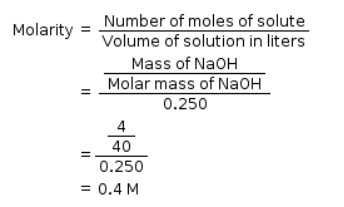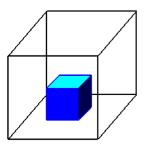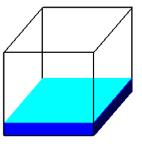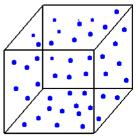Courses

# Test: Basic Concepts Of Chemistry

## 25 Questions MCQ Test Chemistry Class 11 | Test: Basic Concepts Of Chemistry

Description
This mock test of Test: Basic Concepts Of Chemistry for Class 11 helps you for every Class 11 entrance exam. This contains 25 Multiple Choice Questions for Class 11 Test: Basic Concepts Of Chemistry (mcq) to study with solutions a complete question bank. The solved questions answers in this Test: Basic Concepts Of Chemistry quiz give you a good mix of easy questions and tough questions. Class 11 students definitely take this Test: Basic Concepts Of Chemistry exercise for a better result in the exam. You can find other Test: Basic Concepts Of Chemistry extra questions, long questions & short questions for Class 11 on EduRev as well by searching above.
QUESTION: 1

### Physical properties are those properties which ______ measured or observed ______ changing the identity or the composition of the substance

Solution:

Physical properties are those which can be measured or observed without changing the identity or composition of the substance.

QUESTION: 2

### According to the law of conservation of mass, a balanced chemical equation has

Solution:

According to this total mass of reactants = total mass of product so no. of atoms of each element in reactant is equal to no. of atoms of that element in product

QUESTION: 3

### The molar mass of Al2O3 is

Solution:

Molar mass = 2(27) +3 (16) = 102g.

QUESTION: 4

For the reaction
Fe2 O3 (s) + 3 CO (g) → 2 Fe (g) + 3 CO2,
224 g of CO is available to react with 400 g Fe2O3, the yield of iron and CO2, are:

Solution:

Moles of CO =8 moles Moles of Fe2O3= 2.5 moles.

3 moles of CO is needed for 1 mole of Fe2O3 so 8 moles of CO will require 2.66 mole of Fe2O3 so Fe2O3 is limiting reagent.

1 mole of Fe2O3 produce 2 mole of Fe so 2.5 mole of Fe2O3will produce 5 mole of Fe = 280g of Fe.

Also 1 mole of Fe2O3 also produce 3 mole of CO2 so 2.5 mole of Fe2O3 will produce 7.5 mole of CO2=330g.

QUESTION: 5

SI units for Base Physical Quantities of length, mass and current are

Solution:

m,Kg, A are SI units.

QUESTION: 6

Molarity is defined as

Solution:

Molarity is defined as no. of moles of solute present per litre of solution.

QUESTION: 7

The molar mass of C6H10O is

Solution:

Molar mass = 6(12) + 10(1) + 5(16) = 162g

QUESTION: 8

A measured temperature is 1000F on Fahrenheit scale, then what is this reading be on Celsius scale:

Solution:

F = 9/50c + 32 Here F=100
so 100=9/50c)+32 9/50c)=100 - 32 = 68 C = 68*5/9 = 37.75
= 37.8C

QUESTION: 9

SI unit of density is

Solution:

Density = mass/volume. SI unit of mass is kg and that of volume is m3

QUESTION: 10

The calculation of masses (sometimes volumes also) of the reactants and the products involved in a chemical reaction is called

Solution:

The calculation of masses (sometimes volumes also) of the reactants and the products involved in a chemical reaction is called stoichiometry.

QUESTION: 11

The molar mass of CaCO3 is

Solution:

Molar mass = 40 + 12 + 3(16) = 100g

QUESTION: 12

There are ____ in 12.0 ml?

Solution:

Since we know that 1litre -1000ml 1ml-1\1000litre So,12ml-1/1000×12=12/1000 =0.012

QUESTION: 13

Molarity of NaOH in a solution prepared by dissolving 4 g of NaOH in enough water to form 250 ml of solution is:

Solution:QUESTION: 14

The kelvin scale is related to celsius scale by

Solution:

K = °C + 273.15

QUESTION: 15

Molecular mass of glucose (C6H12O6) is

Solution:

Molecular mass will be = 6(12) + 12(1) + 6(16) = 180u.

QUESTION: 16

The molar mass of AgNO3 is

Solution:

Molar mass of AgNO= mass of Ag + N + O3 = 107.87 + 14 + 3* 16 = 107. 87 + 14 + 48 = 169.87 g

QUESTION: 17

The molar mass of ZnSO4 is

Solution:

Chemical Formula: ZnSO4
Molar Mass: 161.47 g/mol (anhydrous)

QUESTION: 18

How many atoms of hydrogen are in 67.2 L of H2 at STP?

Solution:

One mole of any gas at STP has a volume of 22.4 L. So first determine the number of moles of gas you have.

67.2/22.4 = 3 moles of H2

One mole of any substance contains Avogadro's number of atoms = 6.022 × 1023 atoms.

So multiply number of moles × number of atoms/mole = 1.8066 × 1024 atoms of H2.

QUESTION: 19

In scientific notation for such numbers, any number can be represented in the form N × 10n where

Solution:

n can vary from to 10 and can be +ve or –ve.

QUESTION: 20

Choose the most appropriate answer amongst the options given below for the statement.

A solution of a desired concentration is prepared by diluting

Solution:

Stock solution is diluted to prepare the solution of desired concentration.

QUESTION: 21

How many atoms of Oxygen are there in 18g of water?

(Hint: Avogadro’s Number = 6.02 x 1023 atoms/mol)

Solution:

18g H2O = 1mol water = 6.02 x 1023 molecules of water = 6.02 x 1023 atoms of oxygen.

QUESTION: 22

Chemistry does not play a major role in

Solution:

Chemistry does not deal in explaining superconductivity.

QUESTION: 23

If a matter has definite volume and definite shape, then it is:

Solution:Solid
Holds shape
Fixed VolumeLiquid
Shape of Container
Free Surface
Fixed VolumeGas
Shape Of Container
Volume of Container

Explanation:

Solid is the only state of matter that has a definite shape and definite volume.

QUESTION: 24

The number of moles of solute present in 1 kg of solvent is called:

Solution:

Molality is defined as no. of moles of solute present per kg of solvent.

QUESTION: 25

There are ____ in 0.05 ml?

Solution:

1L=1000mL so 0.00005L=0.05mL.